User’s Guide : Multiple Equation Analysis : Mixed Frequency VAR : Technical Background

Technical Background
For notational simplicity we will assume a mixed frequency VAR model with no constant or exogenous variables. We also assume only two frequencies of data, low and high, and that there are m high frequency periods per low frequency period.
The VAR contains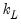variables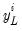, observed at low frequency and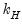variables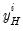observed at the high frequency. Let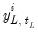represent the i-th low frequency variable observed during low-frequency period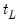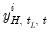represent the i-th high frequency variable observed in the t-th high-frequency period during low-frequency periodStacking theandvariables into the matrices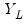and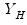, respectively, and for brevity ignoring the intercepts and exogenous variables, we may write the VAR as: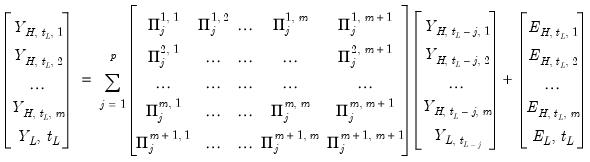(48.1)
where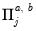is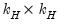,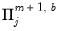is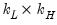, and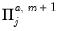is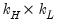, for all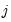,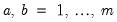, and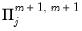is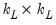.
The U-MIDAS approach constructs these data and simply uses classical VAR least squares to estimate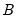, the stacked matrix of coefficients.
Bayesian mixed-frequency VAR estimation (Ghysels 2016), analogously to Bayesian VAR estimation, requires you to specify prior distributions for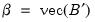and the residual covariance matrix,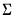( “Technical Background”).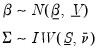(48.2)
These priors coupled with the standard likelihood for a VAR model yields conditional posteriors for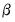andof: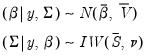(48.3)(48.4)
where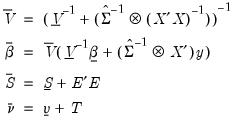(48.5)
The prior onis constructed like the Litterman prior in standard Bayesian VARs.
As with Litterman, the prior mean ofis assumed to be all zero, other than the own first lag terms. However the Ghysels prior differs slightly by allowing a different hyper-parameter for the own lag term depending on whether a variable is high or low frequency. Another crucial difference is that the variables are assumed to follow an AR(1) process in the high-frequency, so that when estimating in low-frequency space, the parameter is exponential.
The prior variance ofis similar to a Litterman prior, but with subtle differences to allow for the cross-frequency variances.
Thus, for the coefficient priors we have:

 Element Mean Variance Dimension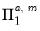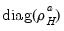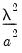,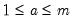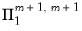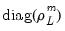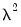0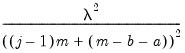,,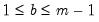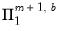0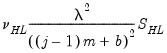,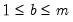0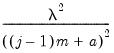,,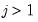0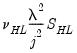,0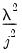,where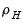,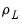,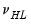and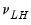are hyper-parameters, and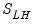is amatrix with the (i,j)-th element equal to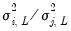,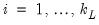,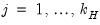where the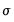are taken from a univariate AR(1) estimation.
The covariance term between the i-th endogenous variable and an exogenous variable is set to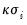.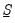is formed by taking a scaled identity matrix or by scaling an initial estimate of the variance taken from a classical least squares U-MIDAS estimation: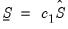(48.6)
where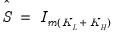or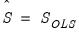.